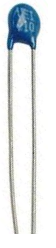﻿ How to Test a Thermistor ﻿# How to Test a ThermistorIn this article, we go over how to test a thermistor- to check whether it is good or not.

A thermistor is a resistor whose resistance changes according to the temperature it is exposed to.

There are 2 main types of thermistors: negative temperature coefficient (NTC) thermistors and positive temperature coefficient (PTC) thermistors.

NTC thermistors are thermistors whose resistance decreases when the temperature it is exposed to increases. So if heat is applied to a NTC thermistor, its resistance will drop accordingly. PTC thermistors are thermistors whose resistance increases when the temperature it is exposed to increases. So if heat is applied to a PTC thermistor, its resistance increases accordingly.

Being that thermistors are components which vary their resistance according to the amount of heat applied, we can exploit this property to test it.

All we need to test a thermistor is an ohmmeter or a multimeter that contains an ohmmeter. We then use the ohmmeter to check the resistance after various stages of our testing to see if the thermistor is behaving according to the characteristics of the thermistor. If it is, it is good. If it does not, then it is faulty.

Equipment Needed to Test a Thermistor

• Thermistor (to be tested)
• Heater, dry blower, or any type of heating device

### How to Test an NTC Thermistor

An NTC thermistor, again, is a thermistors whose resistance decreases when the temperature it is exposed to increases.

We will check this characteristic to see whether it behaves accordingly.

First, before applying any heat to the thermistor, check its rated value. For example, the thermistor may be 100Ω, 1KΩ, 10KΩ, 100KΩ, etc.

Before any heat is applied to the thermistor, its resistance value should be very closely near its rated resistance value.

So, for example, if we are testing a 10KΩ thermistor, before we apply any heat, its resistance value should be very close to 10KΩ. If you're in a relatively warm room, it may be about 9.3KΩ. If you're in a colder area, it will be at a higher value.This is the first preliminary test to check whether a thermistor is good. If it is near its rated resistance value, then so far, it is good. If it is very far or much different than its rated value, then the thermistor is faulty and should be discarded.

The next test is to apply heat to the thermistor. This can be done either by a heater, dry blower, or any type of heating device.When you apply heat to the body of a thermistor, its resistance should begin to steadily decline within seconds after heat is applied. If the NTC thermistor follows this behavior, then it is good. If it does not, it is faulty and should be discarded.

### How to Test a PTC Thermistor

Testing a PTC thermistor follows all the same steps as a NTC thermistor.

First check, with an ohmmeter, the thermistor without any heat applied. With a PTC thermistor at room temperature or below, the thermistor should measure a very low resistance.

Now apply heat, just like before, to the thermistor's body. The resistance of the PTC thermistor should gradually and steadily rise, within seconds of being applied.

If the resistance increases steadily, then the PTC thermistor is good. If it does not, it is faulty and should be discarded.

Related Resources

How to Build Simple Thermistor Circuits

What is Sensible Heat?